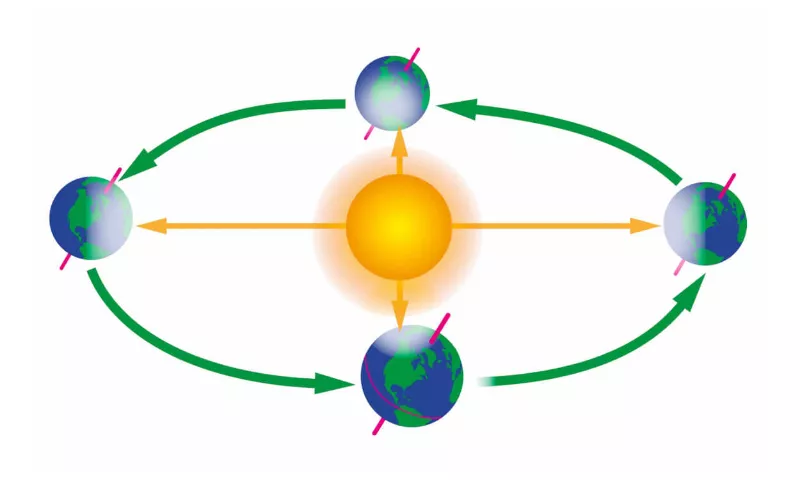Earth Spin

Right now, you are traveling through space at about 67,000 MPH. Earth spins at about 1,000 miles per hour and is currently moving through space at about 67,000 miles per hour around the sun.Share

Earth's spin is constant, but the speed depends on what latitude you are located at. Here's an example. The circumference (the distance around the largest part of the Earth) is roughly 24,898 miles (40,070 kilometers), according to NASA. (This area is also called the equator.) If you estimate that a day is 24 hours long, you divide the circumference by the length of the day. This produces a speed at the equator of about 1,037 mph (1,670 km/h).
?
Earth's spin, of course, is not the only motion we have in space. Our orbital speed around the sun is about 67,000 mph (107,000 km/h), according to Cornell. We can calculate that with basic geometry.
First, we have to figure out how far Earth travels. Earth takes about 365 days to orbit the sun. The orbit is an ellipse, but to make the math simpler, let's say it's a circle. So, Earth's orbit is the circumference of a circle. The distance from Earth to the sun — called an astronomical unit— is 92,955,807 miles (149,597,870 kilometers), according to the International Astronomers Union. That is the radius (r). The circumference of a circle is equal to 2 x ? x r. So in one year, Earth travels about 584 million miles (940 million km).
Since speed is equal to the distance traveled over the time is taken, Earth's speed is calculated by dividing 584 million miles (940 million km) by­­ 365.25 days and dividing that result by 24 hours to get miles per hour or km per hour. So, Earth travels about 1.6 million miles (2.6 million km) a day, or 66,627 mph (107,226 km/h).

• You Might Also Like
•Earth's oceans are so large and deep, humans have only explored 5% of them.The Earth, seen from the moon, also goes through phases.In 1815, Mount Tambora in Indonesia erupted (believed to be the largest eruption of all time), creating a crater on its top 2,000 feet deep after it blew off 4,000 feet of the mountain.